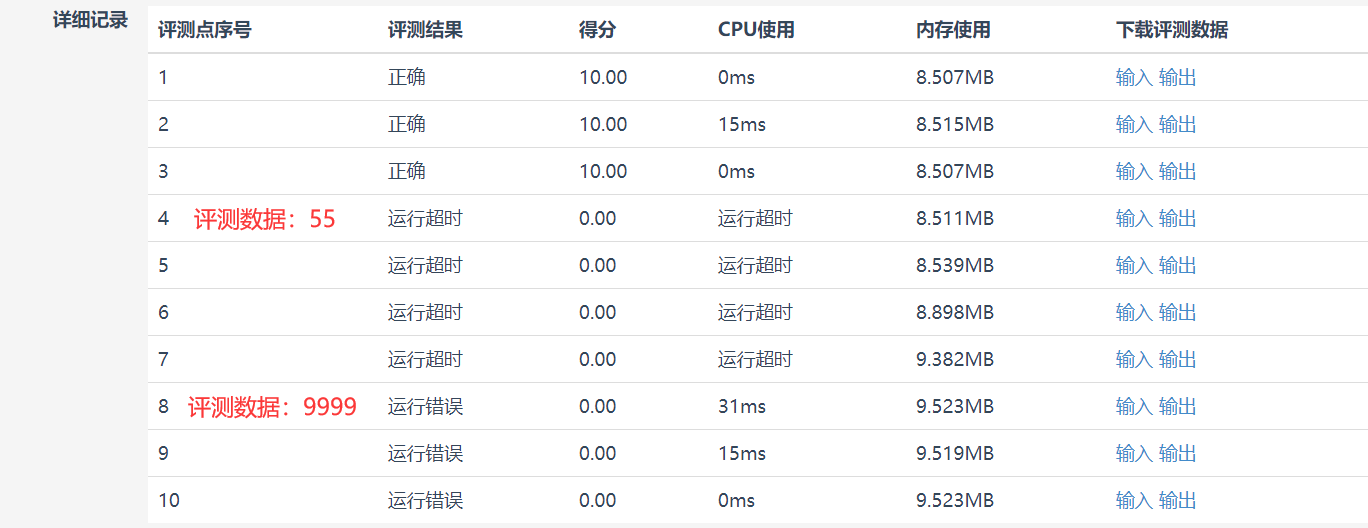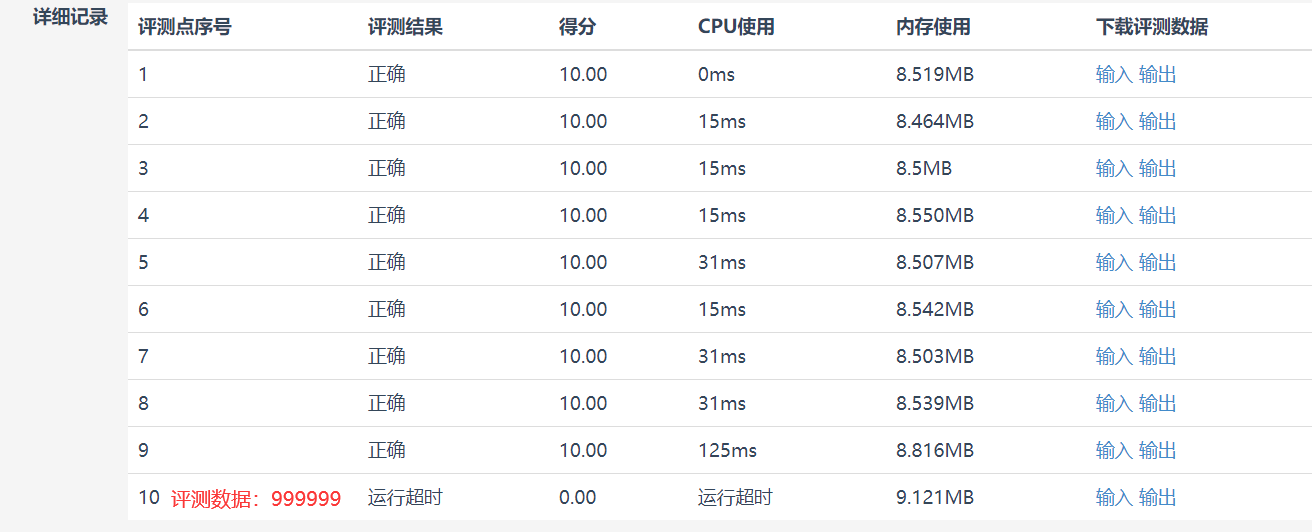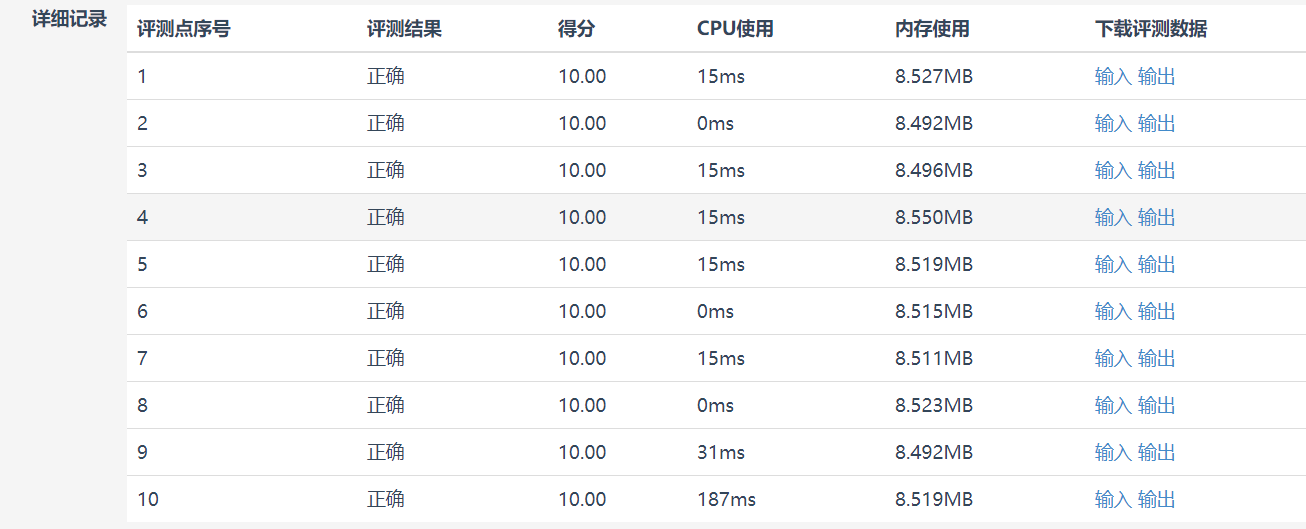• 斐波那契数列python
2022-01-24 19:05:59

感觉网上有些代码有点复杂，整理了斐波那契数列的递推和递归两种写法。都是输入n之后，答案给出第n个数是几。

递推代码

python

fib = [0 for i in range(100000)]
n = int(input())
def fibo():
fib,fib = 1,1
for i in range(2,n):
fib[i] = fib[i-1] + fib[i-2]
print(fib[n-1])
fibo()

递归代码

python

n = int(input())
def fibo(n):
if n<=2:
return 1
else:
return(fibo(n-1)+fibo(n-2))
print(fibo(n))

记忆化+递归

n = int(input())
data = [0 for i in range(100)]
def fibo(n):
if n<=2:
data[n] = 1
return data[n]
if data[n] != 0:
return data[n]
data[n] = fibo(n-1)+fibo(n-2)
return data[n]
print(fibo(n))python 开发语言
更多相关内容
• 第4篇 斐波那契数列python实现 知识点：递归和循环 要求 大家都知道斐波那契数列，现在要求输入一个整数n，请你输出斐波那契数列的第n项。 n<=39 斐波那契数列的定义： F(0)=0，F(1)=1, F(n)=F(n-1)+F(n-2)（n>=2...
•python编程
• 计算斐波那契数列。具体什么是斐波那契数列，那就是0，1，1，2，3，5，8，13，21，34，55，89，144，233。 要求： 时间复杂度尽可能少 分析： 给出了三种方法： 方法1：递归的方法，在这里空间复杂度非常大。...
• 斐波那契数列就是从第三位开始，每一位等于前两项之和。 前两位单独输出，从第三位开始利用for循环解决（当然也可以通过在for循环中增加判断语句的方式，不采用单独输出，但是每次循环都会调用if语句会增加运行时长...

【问题思路】
斐波那契数列就是从第三位开始，每一位等于前两项之和。 前两位单独输出，从第三位开始利用for循环解决（当然也可以通过在for循环中增加判断语句的方式，不采用单独输出，但是每次循环都会调用if语句会增加运行时长）。
【Python代码】

#斐波那契数列
num = int(input("请输入要输出的数列的项数："))
Fib1 = 1
Fib2 = 1
print('{:>8d}'.format(Fib1),end=" ")
print('{:>8d}'.format(Fib2),end=" ")
n=2
for i in range(3,num+1):
Fibi = Fib1+Fib2
Fib1=Fib2
Fib2=Fibi
n+=1
#每个数占8位且右对齐，每五个换行
print('{:>8d}'.format(Fibi),end=" ")
if n%5==0 and n!=num:
print('\n')


【测试样例】展开全文python
• 主要介绍了python3实现斐波那契数列(4种方法)，文中通过示例代码介绍的非常详细，对大家的学习或者工作具有一定的参考学习价值，需要的朋友们下面随着小编来一起学习学习吧
• 蓝桥杯 入门训练 Fibonacci数列Python 实现）

## 问题描述

Fibonacci数列的递推公式为：Fn=Fn-1+Fn-2，其中F1=F2=1。

当n比较大时，Fn也非常大，现在我们想知道，Fn除以10007的余数是多少。

输入格式
输入包含一个整数n。

输出格式
输出一行，包含一个整数，表示Fn除以10007的余数。

说明：在本题中，答案是要求Fn除以10007的余数，因此我们***只要能算出这个余数即可***，而不需要先计算出Fn的准确值，再将计算的结果除以10007取余数，直接计算余数往往比先算出原数再取余简单。

样例输入
10
样例输出
55
样例输入
22
样例输出
7704

数据规模与约定
1 <= n <= 1,000,000

## 第一次的代码

总是超时，还出错：

n = int(input())
def f(num) :
if num == 1 or num == 2 :
return 1
return f(num-1) + f(num-2)
a = f(n)
result = a % 10007
print(result)运行错误：RecursionError: maximum recursion depth exceeded in comparison（超过了最大递归深度）

原因：由于大多数时候我们在实现斐波拉契数列的时候都是用的递归，可能会只想着优化递归的写法，递归的时间复杂度确实很低但是不适合本题要求的“计算Fn的余数”

## 第二次的代码：

n = int(input())
res1, res2 = 1, 1
for i in range(3, n+1):
res1, res2 = res2, (res1 + res2)
print(res2 % 10007)可以看到在测试999999的时候，显示超时。这时候我们要注意到题目给我们的说明“只要能算出这个余数即可，而不需要先计算出Fn的准确值”。

## 第三次的代码：

n = int(input())
res1, res2 = 1, 1
for i in range(3, n+1):
res1, res2 = res2 % 10007, (res1 + res2) % 10007
print(res2)## 总结：

循环的时间复杂度和空间复杂度都优于用 递归实现。
递归的优越性在于条理清晰，可读性强，比较适宜于问题本身是递归性质的、用循环难于解决的问题。在二者都不难的情况下，一般都是优先选用循环来解决问题的。

展开全文python
• 斐波那契数列Fibonacci sequence），又称黄金分割数列、因数学家列昂纳多·斐波那契（Leonardoda Fibonacci）以兔子繁殖为例子而引入，故又称为“兔子数列”，指的是这样一个数列：1、1、2、3、5、8、13、21、34、...
• 输出斐波那契数列”"" if n <= 1: return n else: return(recur_fibo(n-1) + recur_fibo(n-2)) 获取用户输入 nterms = int(input("您要输出几项? ")) 检查输入的数字是否正确 if nterms <= 0: print...
def recur_fibo(n):
"""递归函数
输出斐波那契数列"""
if n <= 1:
return n
else:
return(recur_fibo(n-1) + recur_fibo(n-2))
# 获取用户输入
nterms = int(input("您要输出几项? "))
# 检查输入的数字是否正确
if nterms <= 0:
print("输入正数")
else:
print("斐波那契数列:")
for i in range(nterms):
print(recur_fibo(i))
展开全文• 斐波那契数列即 1,1,2,...,f(n)=f(n-1) + f(n-2) 关于斐波那契数列的实现，最简单的一种，大家都会想到： def fib1(n): if n==0 or n==1: return 1 else: return fib1(n-1) + fib1(n-2) print（fib1(n)） ...
• Fibonacci数列的递推公式为：Fn=Fn-1+Fn-2，其中F1=F2=1。 当n比较大时，Fn也非常大，现在我们想知道，Fn除以10007的余数是多少。 输入格式 输入包含一个整数n。 输出格式 输出一行，包含一个整数，表示Fn除以...python 算法
• 斐波那契数列Fibonacci sequence），又称黄金分割数列、因数学家列昂纳多·斐波那契（Leonardoda Fibonacci）以兔子繁殖为例子而引入，故又称为“兔子数列”，指的是这样一个数列：1、1、2、3、5、8、13、21、34、...
• 斐波那契数列Fibonacci sequence），又称黄金分割数列、因数学家列昂纳多·斐波那契（Leonardoda Fibonacci）以兔子繁殖为例子而引入，故又称为“兔子数列”，指的是这样一个数列：1、1、2、3、5、8、13、21、34、...斐波那契数列 算法 递归
• 1，1，2，3，5，8，13，21，34，55，89...... ...斐波那契数列／兔子数列.jpg 在此之前，我都是只知道“1，1，2，3，5，8......”这个数字序列，并不知道它的名字，也不知道它从哪里来。如果我是这个序列，我一定很...
• 斐波那契数列Fibonacci sequence），又称黄金分割数列、因数学家莱昂纳多·斐波那契（Leonardoda Fibonacci）以兔子繁殖为例子而引入，故又称为“兔子数列”，指的是这样一个数列：0、1、1、2、3、5、8、13、21、...python 算法
• １.斐波那契问题最简单的是递归实现，python3默认最大递归深度为998,可以通过sys.setrecursionlimit(设置的次数)来设置最大递归深度,但是递归效率太低,所以不推荐,仅供了解 def feibonacci(n): if n <=2: ...
• 1.斐波那契数列 题目: 斐波那契数列：1, 2, 3, 5, 8, 13, 21.....根据这样的规律，编程求出400万以内最大的斐波那契数，并求出他是第几个斐波那契数 解题思路: 首先要得到前两项,从第三项开始,每一项都为前两项...算法 Python
• Fibonacci数列的递推公式为：Fn=Fn-1+Fn-2，其中F1=F2=1。 当n比较大时，Fn也非常大，现在我们想知道，Fn除以10007的余数是多少。 输入格式 输入包含一个整数n。 输出格式 输出一行，包含一个整数，表示Fn除以10007...python
• 剑指offer（10）——斐波那契数列 python 实现，最后有彩蛋一、题目描述二、解题方法（1）不得分的常规递归方法实现：（2）往后推移法（3）使用生成器（具备生成器的优点，需要的时候再生成）彩蛋 一、题目描述 写...剑指offer 斐波那契数列 Python
•python 开发语言 算法
• 方式1：直接递归。 缺点：超出时间限制。。。 class Solution: def fib(self, n: int) ->...方式2：利用python的list做存储，自底向上Bottum-up 缺点：耗时依然长 class Solution: def fileetcode python 数据结构 算法
• 本文以一个简单的实例讲述了python实现斐波那契数列数列递归函数的方法，代码精简易懂。分享给大家供大家参考之用。 主要函数代码如下： def fab(n): if n==1: return 1 if n==0: return 0 else: result=int...
• 斐波那契数列Fibonacci）最早由印度数学家Gopala提出，而第一个真正研究斐波那契数列的是意大利数学家 Leonardo Fibonacci斐波那契数列的定义很简单，用数学函数可表示为： 数列从0和1开始，之后的数由前两个数...
• 试题 基础练习 Fibonacci数列 资源限制 时间限制：1.0s 内存限制：256.0MB 问题描述 Fibonacci数列的递推公式为：Fn=Fn-1+Fn-2，其中F1=F2=1。 当n比较大时，Fn也非常大，现在我们想知道，Fn除以10007的余数是...python 算法
• Fibonacci为1200年代的欧洲数学家，在他的着作中曾经提到：若有一只兔子每个月生一只小兔子，一个月后也开 始生产。起初只有一只兔子，一个月后就有两只兔子，二个月后就有三只兔子，三个月后有五只兔子…… 数列...斐波那契数列 Python...